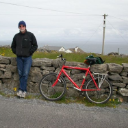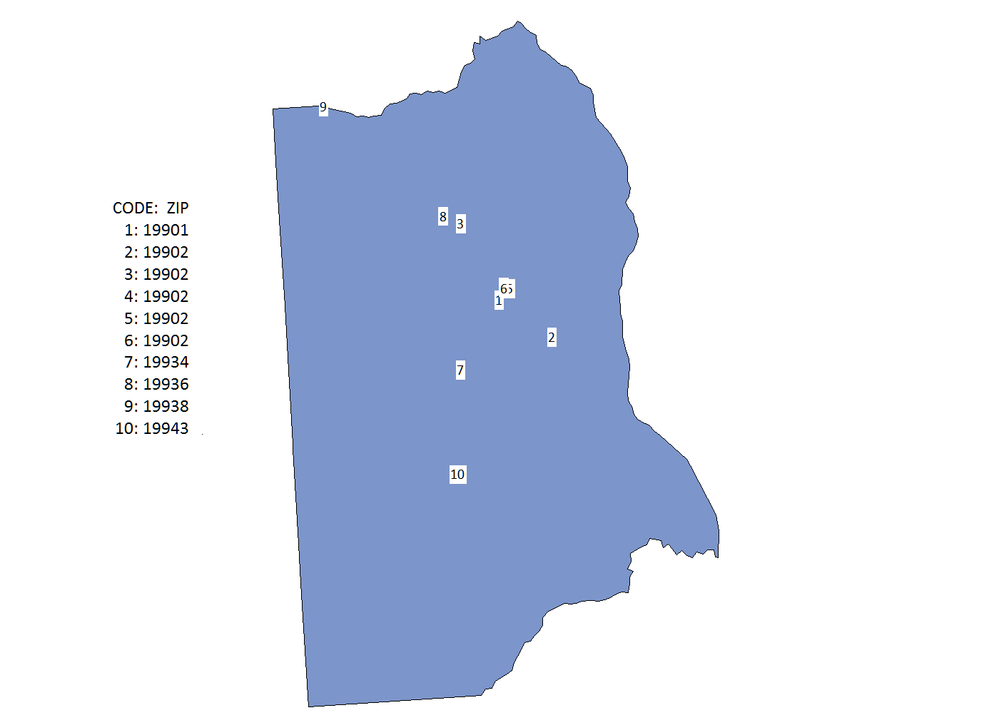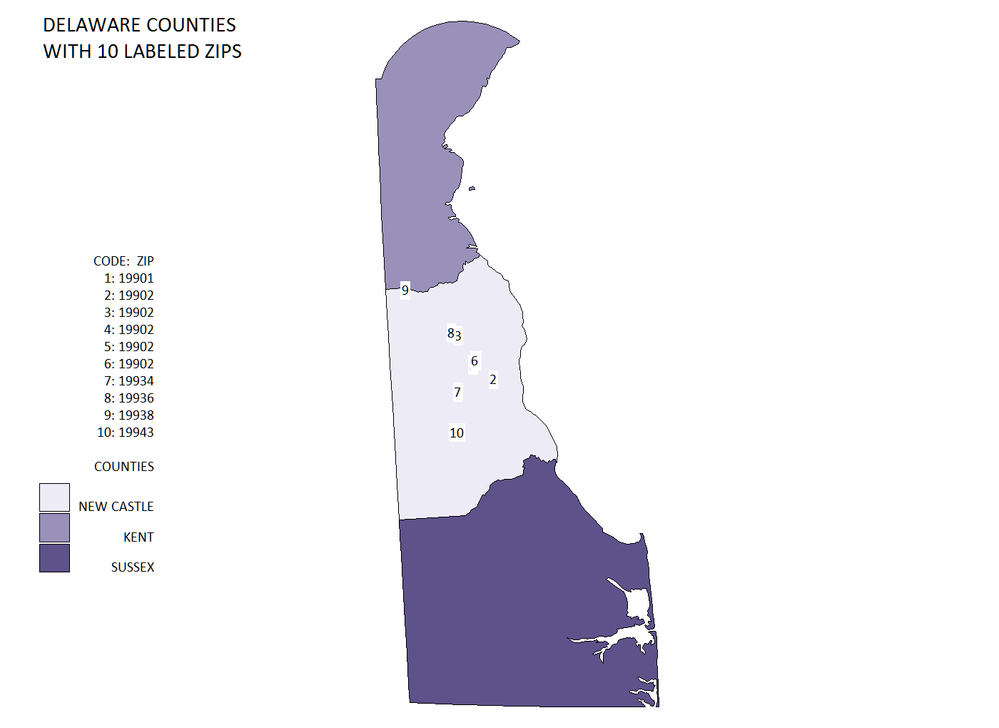## Numbered Marking on a Map

I am making a map and have twenty different points I would like to mark. Right now I have a star marking each point. Is there a way for me to use mark each point using a corresponding number instead of the star?

Thanks.

10 REPLIES 10

## Numbered Marking on a Map

Are you using Proc Gmap to draw the map, and placing the stars on it with annotate?

If so .... then you can put any text you want (such as a number) in the text variable, instead of the star.  You'll probably also need to change the style= variable (it's probably something like style='marker', and you'll probably want to change it to style='albany amt' ... or leave out the style variable and let it default).

## Numbered Marking on a Map

Yes I am using Proc Gmap and annotate. Thanks for your help.

## Numbered Marking on a Map

I am having trouble again I need the marker to go up from 1 to 20. How can I do this?

## Numbered Marking on a Map

Could you explain a little more?

I can't quite picture in my mind what you're wanting.

## Numbered Marking on a Map

My bad about being a little vague. I have a map and I have 20 buildings with different names, where at the moment I have all of them marked by the number "1" on the map. What I am looking for as my end result is to have a key off to the side of the map with the buildings names and a number associated with the building, 1 through 20. These numbers would coincide with the number on the map showing where the buildings are. Thanks.

## Numbered Marking on a Map

Ahh! - ok...

So, presumably you have an annotate data set, with X & Y variables containing the location you want each number/label (formerly 'star') at.  And you are annotating this text with the annotate function='label'.  If the value is currently coming out as a '1', that indicates that the value in your "text" variable is '1'.

You'll want to set your text variable to the values you want annotated on the map.

Here's an over-simplified pseudo-example...

x=23; y=47; text='1';

x=34; y=12; text='2';

x=87; y=43; text='3';

I recommend doing a "proc print" of your annotate data, or look at it with the table viewer.

## Numbered Marking on a Map

Thanks a lot for your help. I am assuming that this long text variable will be put inbetween style and color, right?MikeZdeb
Rhodochrosite | Level 12

## Re: Numbered Marking on a Map

hi ... you can add that number:building name key with a legend

here's an example that uses one county in Delaware with 10 zip centroid locations added

data points (drop=state county);

retain xsys ysys '2' hsys '3' size 2 function 'label' style 'calibri' cbox 'white' when 'a';

set sashelp.zipcode (keep=state county x y zip obs=10);

where state eq 10 and county eq 1;

x = -x * constant('pi') / 180;

y = y * constant('pi') / 180;

text = cat(_n_);

run;

data both;

set maps.counties (where=(state eq 10 and county eq 1)) points;

run;

proc gproject data=both out=proj;

id county;

run;

goptions reset=all ftext='calibri' htext=3 gunit=pct;

legend1 mode=share origin=(2,40)pct value=none shape=bar(.00001,.00001)pct

label=(j=r 'CODE:  ZIP' j=r '1: 19901' j=r '2: 19902' j=r '3: 19902' j=r '4: 19902' j=r '5: 19902'

j=r '6: 19902' j=r '7: 19934' j=r '8: 19936' j=r '9: 19938' j=r '10: 19943');

proc gmap data=proj(obs=1) map=proj (where=(segment)) anno=proj (where=(^segment));

id county;

choro county / legend = legend1;

run;

quit;## Numbered Marking on a Map

Is there a way to put two legends on the map?MikeZdeb
Rhodochrosite | Level 12

## Re: Numbered Marking on a Map

hi ... don't think so, but the LEGEND can still be used to give info on map areas, for example ...

data points (drop=state county);

retain xsys ysys '2' hsys '3' size 2 function 'label' style 'calibri' cbox 'white' when 'a';

set sashelp.zipcode (keep=state county x y zip obs=10);

where state eq 10 and county eq 1;

x = -x * constant('pi') / 180;

y = y * constant('pi') / 180;

text = cat(_n_);

run;

* all Delaware counties used;

data both;

set maps.counties (where=(state eq 10)) points;

run;

proc gproject data=both out=proj;

id county;

run;

goptions reset=all ftext='calibri' htext=2 gunit=pct;

* legend now includes both the ZIP labels and symbols (bars) for map areas;

legend1 mode=share origin=(2,20)pct across=1 shape=bar(3,4)pct

value=(j=r 'KENT' j=r 'NEW CASTLE' j=r 'SUSSEX')

label=(position=top j=r 'CODE:  ZIP' j=r '1: 19901' j=r '2: 19902' j=r '3: 19902' j=r '4: 19902' j=r '5: 19902'

j=r '6: 19902' j=r '7: 19934' j=r '8: 19936' j=r '9: 19938' j=r '10: 19943'

j=r " " j=r 'COUNTIES');

* some white space on the left;

title1 a=90 ls=2;

proc gmap data=proj map=proj (where=(segment)) anno=proj (where=(^segment));

id county;

choro county / levels=3 legend=legend1;

note h=3 j=l 'DELAWARE COUNTIES' j=l 'WITH 10 LABELED ZIPS';

run;

quit;Discussion stats
• 10 replies
• 1443 views
• 0 likes
• 3 in conversation